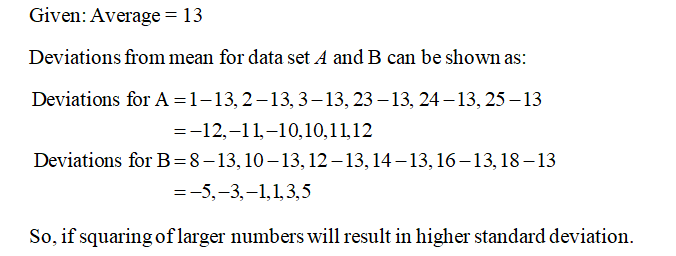# Both sets of values have an average of 13. Is Set A's standard deviation smaller, larger, or about the same as Set B's? (Note: This question can be answered by knowing the concept of standard deviation, without actually computing the standard deviation).Set A: 1 2 3 23 24 25Set B: 8 10 12 14 16 18

Question
1 views

Both sets of values have an average of 13. Is Set A's standard deviation smaller, larger, or about the same as Set B's? (Note: This question can be answered by knowing the concept of standard deviation, without actually computing the standard deviation).

Set A: 1 2 3 23 24 25

Set B: 8 10 12 14 16 18

check_circle

Step 1...

### Want to see the full answer?

See Solution

#### Want to see this answer and more?

Solutions are written by subject experts who are available 24/7. Questions are typically answered within 1 hour.*

See Solution
*Response times may vary by subject and question.
Tagged in